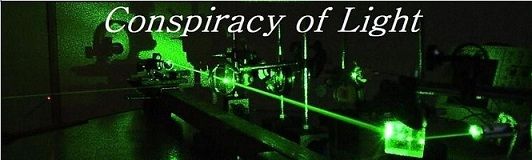The search for  new physics.

Spacetime- Right or Wrong?

Doug Marett 2013

Einstein’s concept for space-time calls for 4 dimensions of space, three for physical dimensions of space (x,y,z) and one for time (t). The idea is that time is a dimension in the sense that the past, present and future coexist – so different places in time can be defined by simply giving them a coordinate on this space-time dimension. Let’s take the example of the twins paradox. The stay on earth twin (A) has the space-time coordinates x,y,z,t. The twin that leaves the earth on a rocket whose clock initially counted at the same rate as twin A, now has his clock slow due to his velocity v. Thus after some interval in time the traveling twin B has moved in space to a new coordinate x’,y’,z’, but since his clock is now counting slower, he is not in the same time frame as twin A, so at any given time t for twin A, twin B is at some different time , which we will call t’.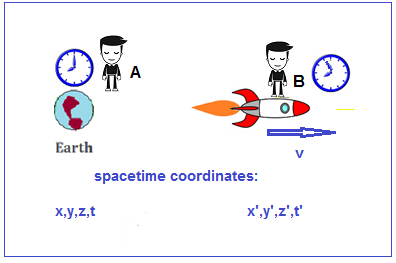The obvious implication is that twin B and his rocket are not only in a different time coordinate that twin A, but are also experiencing time moving more slowly than twin A. Stopwatches held by both twin A and B would count at different rates. If what Einstein proposed is true, we can only conclude that the physical environment of the rocket and twin B is distinct from that of twin A in that they do not share the same rate of real time. Is there any way to test this? One conclusion that logically comes up is that if both twin A and twin B measure the speed of light in their own frames, they will measure them as both being C=C’ = 3E8 m/s. However, if they arrive at the same answer using two stopwatches that are obviously counting at different rates, then C cannot = C’ if both are measured by the same stopwatch.  This would seem to be a contradiction to the constant speed of light hypothesis. Is there something wrong here? Let’s look at one of the most popular explanations for how time dilation occurs using the light clock analogy, and then proceed to a thought experiment test of this idea.

The Light Clock Analogy

When we see a supernova explode in the sky, we do not say “that event just happened”, since we know the light from that event has taken perhaps thousands of years to reach us from some distant point in space. We would say, rather, that the explosion happened thousands of years ago. This is because our judgement of the simultaneity of events is independent of the speed of light. Rather the speed of light might be used to insinuate when an event could have happened in the past, based on the propagation delay. So why then would we believe that the rate of time itself depends on the speed of light? Consider Einstein’s analogy, often reproduced, of a light beam bouncing between two mirrors. In his analogy, two horizontal mirrors face each other and one mirror is spaced above the other by a distance d. A light pulse bounces vertically between the two mirrors as shown on the left.  The time it takes for the pulse of light to do a round trip (from the top mirror to the bottom and back) is twice the distance d divided by the speed of light. This is his light clock. One tick is one return bounce of the light beam.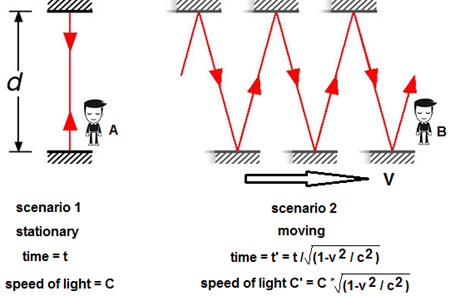Suppose the "light clock" were traveling sideways at a very high (but constant) speed of 0.5 C. Now the pulse would follow the "saw tooth" path shown on the right side of the drawing. Since light speed is the same as before (remember, the speed of light is not changed by the speed of its source), it will take longer to make a round trip. So our "light clock" takes longer to count out its intervals. Another way of saying this is that the clock "ticks" more slowly.

But here lies the problem. This analogy works because the speed of light is constant as seen by observer A. From his vantage point, he can see both clocks working, and although the speed of light is travelling at the same rate in scenario 2, it has taken a longer path to hit the moving mirrors. In taking a longer path, it has taken more time to reach the end. But because we have already said that the rate of time is independent of the speed of light, we cannot assume that “real” time has passed slower for observer B than observer A, we can only conclude that B’s clock has counted mechanically slower because it has taken longer for the light to travel the distance between scenario 2’s mirrors. Now, if observer B measures the speed of light in his moving frame using “real” time, i.e. a clock not affected by the speed of light, he should conclude that the speed of light is slower than C. Observer B is unaware that he is moving, so he perceives the mirrors to be opposite each other and separated by distance d, so he would measure the speed of light to be less than C. He is unaware that from the perspective of observer A, the path d has lengthened. So why does he conclude that the speed of light is instead C? This is because he measures the time elapsed using a clock that is based on the length of time it takes for light to pass between his own moving mirrors. In effect, his moving clock is calibrated by the speed of light seen in his own frame (which is 0.866*C), so consequently, his clock counts slower by exactly this amount (t/0.866). This makes him falsely measure that the light beam to have arrived at velocity C rather than C * 0.866.

Since the slowing of clocks (apparent time dilation) does occur in reality, this implies that the speed of light is only actually C in the stationary frame, and in the moving frame it is actually C * 0.866, but is erroneously measured as being C in his frame due to the slowing error introduced into his moving clock by the longer light path.

If the slowing down of a clock due to a velocity is possibly just an illusion, and real time is unaffected, then perhaps space-time also doesn’t exist in the way Einstein conceived of it. From this revelation we can propose the following conclusion:

The moving frame is not a physical entity with a distinct time t’ ; it is instead only a perspective.

What kind of experiment could we perform to test this idea? Let’s return to the light clock analogy, but this time lets propose to send a laser beam through the stationary observers frame and also the moving observer’s frame together as part of the same light path. If what Einstein says is true, and the moving observer exists in a timeframe distinct from the stationary observer, then the beam returning to the stationary observer through the moving frame should have travelled at some speed slower than C. This is because, as we said earlier, we are now measuring C and C’ with a single stopwatch.

Below is a diagram of the thought experiment. Observer A in the stationary frame fires a laser beam into the moving frame, bouncing off the moving mirrors, and then returns back to his original position, where he measures the elapsed time. Since we know from every laser experiment performed to date that the measured speed of light will always be C, he has no choice but to conclude that the measured speed of light  is still C based on the distance traveled by the beam in his frame.

Light path as observed from the stationary frame by observer A: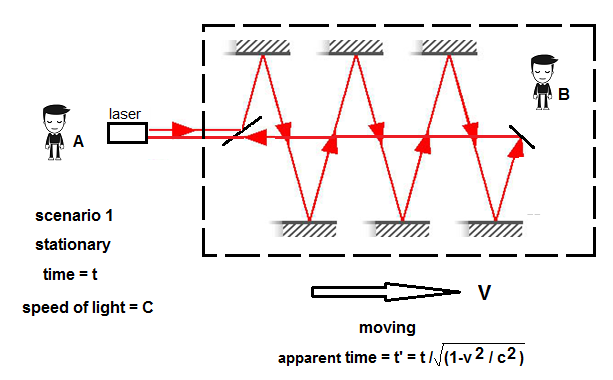The speed of light has not miraculously changed its speed inside of the moving frame, because the frame in motion does not drag its own time with it. The characteristics and rate of time are exactly the same in the stationary frame as in the moving frame - the difference is the lack of agreement by the observers on the distance travelled by the beam of light from each perspective.  Observer B simply sees the beam travel a different path than observer A:

Apparent path traveled by light as seen by the moving observer B: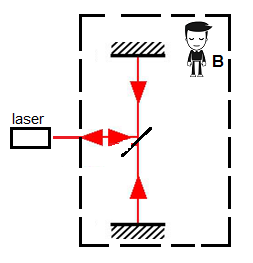The same is true for the stationary and moving clocks –since the clock rates are geared to the speed of light, each clock counts at a different rate because the beam of light has traveled a different distance from each clock’s perspective. Real time has not changed. This matches the known experimental results if and only if the real speed of light is constant in a single frame of reference, the stationary frame.  All other conclusions about the speed of light in the moving frame and the rate of time are illusions caused by the motion of the frame with respect to the preferred, stationary frame for the light beam.

What we can conclude from this exercise is that the slowing of a clock on a rocket ship is meaningless and does not imply that the rate of real time has changed in any frame of reference. It is simply an error of perspective experienced by both the moving observer and the moving clock, in exactly the way that was proposed by Larmor in 1901. This argument should apply to atomic clocks that have been most commonly used to demonstrate time dilation, since a slowing of the speed of light from the perspective of a moving atom should lead to a change in the frequency of emission of the atom. This was in fact the thesis of Herbert Ives when he performed the Ives and Stillwell experiment (Transverse Doppler effect in Canal rays), and he himself states explicitly that he was attempting to prove the theory of Larmor and Lorentz, not Einstein.

But this is not the only reason why Einstein’s concept of space-time must be wrong. What space-time implies is that all events, past, present and future, are already written in the fabric of space. Such a conclusion relegates all human activity to simply being automatons acting out the frames of a preordained film strip. If we were to believe Einstein we would have to believe that there is no free will at all. That in and of itself should be reason enough to reject the space-time concept without further consideration!